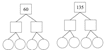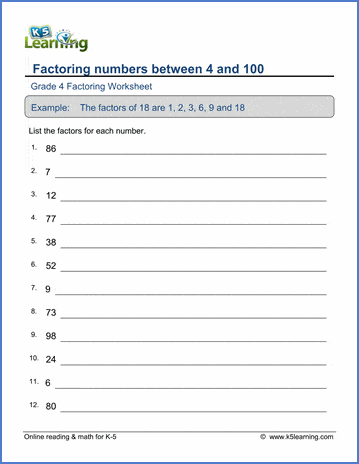# Factoring Worksheets for Grade 4

## Factoring worksheets from K5 Learning

These worksheets ask students to find all the factors of different sized numbers.  Prime factors trees are also introduced. These math worksheets complement our K5 Math online math program.

## Factoring

Example

Find the factors of a number between 4 and 50
6:  1,2,3,6
Find the factors of a number between 4 and 100
52:  1,2,4,13,26,52Prime factors

36:   2 x 2 x 3 x 3
Divisibility rules   Rules for 2,3,5,9,10

## Multiples

Multiples of whole numbers
8:  8, 16, 24, 32, 40